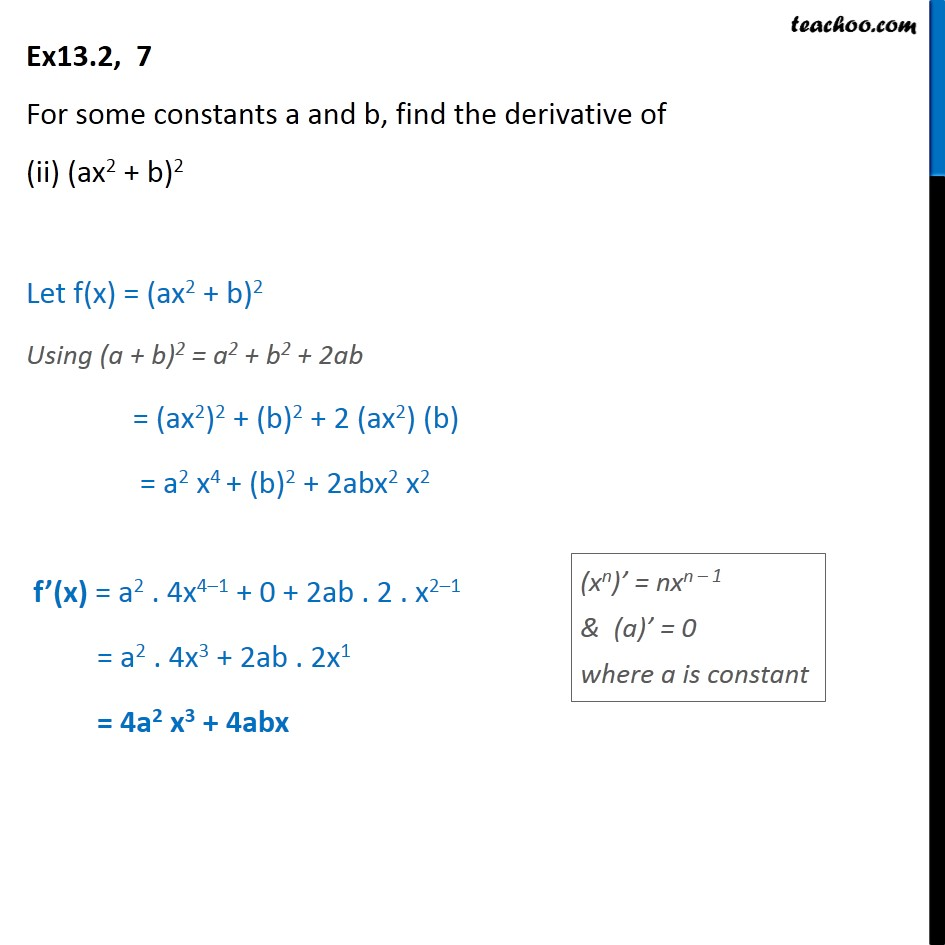1. Chapter 13 Class 11 Limits and Derivatives (Term 1 and Term 2)
2. Serial order wise
3. Ex 13.2 (Term 2)

Transcript

Ex13.2, 7 For some constants a and b, find the derivative of (ii) (ax2 + b)2 Let f(x) = (ax2 + b)2 Using (a + b)2 = a2 + b2 + 2ab = (ax2)2 + (b)2 + 2 (ax2) (b) = a2 x4 + (b)2 + 2abx2 x2 f’(x) = a2 . 4x4–1 + 0 + 2ab . 2 . x2–1 = a2 . 4x3 + 2ab . 2x1 = 4a2 x3 + 4abx